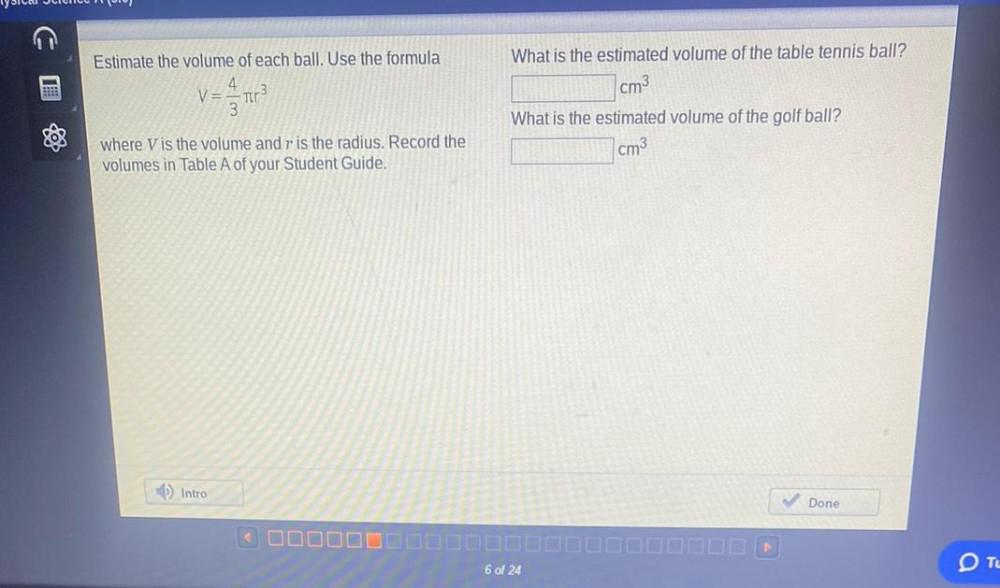Question:

# Estimate the volume of each ball. Use the formula V=4/3πr³ where V is the volume and r is the radius. Record the volumes in Table A of your Student Guide. What is the estimated volume of the table tenEstimate the volume of each ball. Use the formula V=4/3πr³ where V is the volume and r is the radius. Record the volumes in Table A of your Student Guide. What is the estimated volume of the table tennis ball? What is the estimated volume of the golf ball?